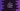# C program to check if a number is Neon or not## C program to check if a number is Neon or not:

In this post, we will learn how to check if a number is a Neon number or not in C programming language. A number is called a Neon number if the sum of the digits of its square value is equal to the number.

For example, 9 is a Neon number because the square of 9 is 81 and 8 + 1 is equal to 9 that is the number.

This program will take a number as input from the user and print one message if it is a Neon Number or not.

## Step to check if a number is Neon or not:

• Take the number as input from the user
• Find the square of the number
• Find the sum of the digits in the square
• Compare the sum with the number. If both are equal then it is a Neon number.

Now let’s write down the program:

### Method 1: C program to check if a number is Neon or not:

``````#include <stdio.h>

int main()
{
int num, square, sumDigits = 0;

printf("Enter a number: ");
scanf("%d", &num);

square = num * num;

while (square != 0)
{
sumDigits += square % 10;
square /= 10;
}

if (num == sumDigits)
{
printf("It is a Neon number\n");
}
else
{
printf("It is not a Neon number\n");
}

return 0;
}``````

In this program,

• num is an integer variable to hold the number
• square is an integer variable to hold a square value
• sumDigits is an integer variable to hold the sum of digits of the square. It is Initialised as 0
• The program is asking the user to enter a number and it stores that number in the num variable
• It finds the square of the number by multiplying the number with itself and stores that value in the square variable
• It uses a while loop to find the sum of digits in the square :

• The while loop will run until the value of square is not equal to zero
• It gets the last digit of square and adds it to sumDigits
• It removes the last digit from the square variable.
• After the loop, it checks if the number is equal to the sum of digits of the square value. If yes, it is a Neon number. Else, it is not.

If you run this program, it will give output as like below:

``````Enter a number: 9
It is a Neon number

Enter a number: 8
It is not a Neon number``````

### Method 2: C program to check if a number is Neon or not by using a different method:

Let’s use a different method to check if a number is Neon or not in C:

``````#include <stdio.h>

int isNeon(int num)
{
int square, sumDigits = 0;
square = num * num;

while (square != 0)
{
sumDigits += square % 10;
square /= 10;
}

return num == sumDigits;
}

int main()
{
int num;

printf("Enter a number: ");
scanf("%d", &num);

if (isNeon(num))
{
printf("It is a Neon number\n");
}
else
{
printf("It is not a Neon number\n");
}

return 0;
}``````

Here,

• We created a new method called isNeon. This method is used to check if a number is Neon or not.

• It takes one integer variable as input and returns an integer.
• It uses the same steps that we did in the last program.
• It returns 1 if the number is Neon, else it returns 0.
• Inside main, we are calling isNeon in the if block. Based on its return value, we are printing a message.

We can use a loop and print all Neon numbers in a given range. Let’s find out all Neon numbers between 0 to 10000.

``````#include <stdio.h>

int isNeon(int num)
{
int square, sumDigits = 0;
square = num * num;

while (square != 0)
{
sumDigits += square % 10;
square /= 10;
}

return num == sumDigits;
}

int main()
{

for (int i = 0; i <= 10000; i++)
{
if (isNeon(i))
{
printf("%d ", i);
}
}

return 0;
}``````

Here,

• We are using the same method isNeon as discussed in the previous example.
• The for loop runs from 0 to 10000. For each value, it calls isNeon to check if it is a Neon number or not. Since we are using i with the loop, it passes i to isNeon.
• If it is a Neon number, it prints that value.

If will print the below output:

``0 1 9``